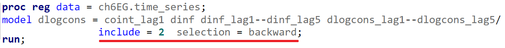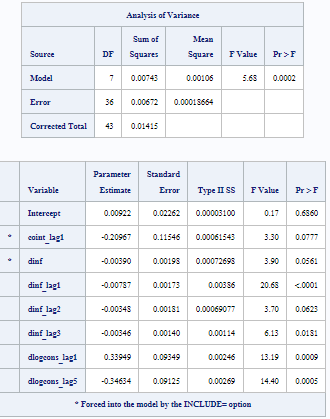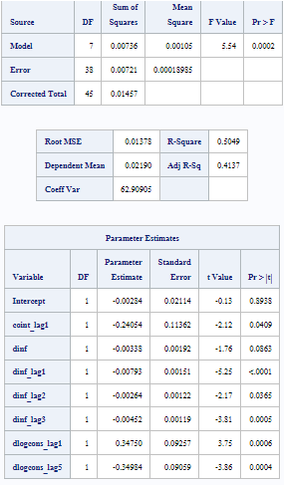## Different output in proc reg

Hello everyone,
I have been using proc reg with backward selection and noticed some discrepancies in my results. I ran the same independent variable in the last step of backward selection and obtained different output. Below, I have included the code and the results. Can someone please explain which output is correct? Thank you.Compared to the below procedure.4 REPLIES 4

## Re: Different output in proc reg

I would conclude from your screen captures that the data set ch6EG.time_series has changed between the two runs. This is apparent because the Corrected Total SS is not the same in the two runs.

--
Paige Miller

Thank You

## Re: Different output in proc reg

Hello @Golf,

It looks like your first model used only 44 observations of the input dataset ch6EG.time_series, whereas the second model used 46 (see column "DF", row "Corrected Total" in the "Analysis of Variance" table or "Number of Observations Used" in the part of the output not shown). The difference is due to two observations having at least one missing value in the variables that have been eliminated in the process of backward selection.

I would prefer the second model because it doesn't seem useful to exclude observations due to missing values in variables that are not used in the model anyway.

Here is a similar example using the SASHELP.CLASS dataset:

``````data class;
set sashelp.class;
if mod(_n_,3) then x=age;
run;

proc reg data=class;
model weight=height x / selection=backward;
quit; /* --> 13 observations used, variable x was removed, leaving Intercept and Height. */

proc reg data=class;
model weight=height;
quit; /* --> Same model, but all 19 observations used, hence different statistics. */``````

## Re: Different output in proc reg

@FreelanceReinh  Thank You.

Discussion stats
• 4 replies
• 280 views
• 1 like
• 3 in conversation